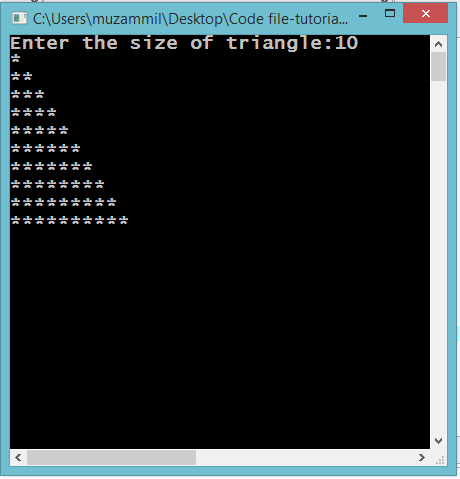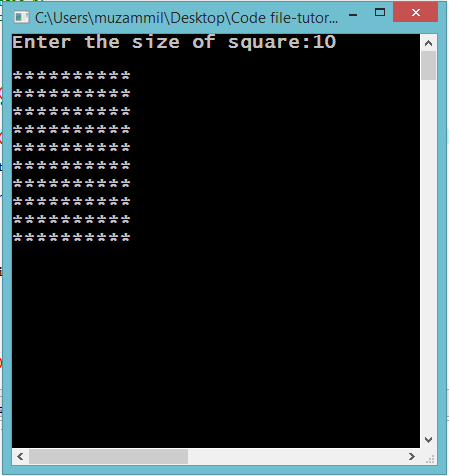# Learning Nested Loops

## Learning Nested loops

In this part you will learn: 1. C syntax 2. Nested Loops 3. More on For loops In this tutorial I will teach you about Nested For loops. We see some more examples of for loop in this tutorial. Printing Triangle with stars Basic Step: Open Dev C++ then File > new > source file and start writing the code below.
`#include<stdio.h>#include<conio.h>`
These two are the most common and basic header files. The first one stdio.h is used to grant us most of the basic functions of C like Input and Output functions. The second one conio.h provides us functions like getch() which is used to pause our screen until we press a button.
`int main(){	int n;	printf("Enter the size of triangle:");	scanf("%d",&n);  	//printf("\n");	for(int i=1;i<=n;i++)	{		for(int j=0;j<i;j++)		{			printf("*");		} 		printf("\n"); 	}   	getch();}`
In this program we are printing a triangle made of stars by taking an input from the user. This input will be the size of triangle. After taking the inputs from the user comes the nested for loops. The first for loop is the one that shifts the line. We initialized its counter with 1 and ran it till the number the number that user entered. The second for loop is the one that prints the stars. It prints as many stars as is the number of the like. For example. If the outer loop is on the second line the inner loop will run twice and print two stars. We started its count from 0 and ran it till the count is less than the count of the outer loop. Note that for every single iteration of the outer loop the inner loop runs completely until it meets the condition. After the inner loop we shifts to the next line by writing a single printf() statement. And when the counter of the outer loop reaches the value which is one greater than ‘n’ it breaks and comes to the function getch(). Afterwards the program ends. Execute > compile then Execute > run OutputPrinting Square with stars Basic Step: Open Dev C++ then File > new > source file and start writing the code below.
`#include<stdio.h>#include<conio.h>`
These two are the most common and basic header files. The first one stdio.h is used to grant us most of the basic functions of C like Input and Output functions. The second one conio.h provides us functions like getch() which is used to pause our screen until we press a button.
`int main(){	int n;	printf("Enter the size of square:");	scanf("%d",&n);   	for(int i=0; i<n ; i++)	{		for(int j=0;j<n;j++)		{			printf("*");		} 		printf("\n"); 	}   	getch();}`
Now in this program we are taking input from the user. That input is a number and size of the square the user wants to print. After taking the input we come to nested for loops. As a square has two dimensions the height and the width. The outer loop is for the height of square while the inner loop is for the width of square. The inner loop runs the same time as the outer loop because the height and the width of a square are always same. Note that for every iteration of the outer loop the inner loop runs ‘n’ times. The inner loop runs a total of n*n times while the outer loop only runs n time. After the inner loop we shifts the line. And then at the end there is the getch() function that pauses are program. Execute > compile then Execute > run Output